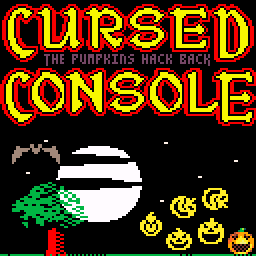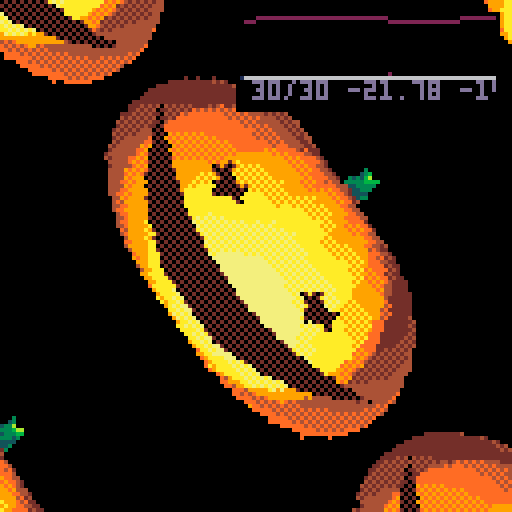### Plot

Oh mighty Zep, a quest for thee: to fix this bug in oh-two-three.
Time's very fabric has been torn, and monsters from the past reborn!
A pumpkin army's impossible roll befouls our once cozy console.

Its humbled clock refunds too much, permitting Cauldron's villain's touch.
Relentlessly they spin and taunt, they dare thee to remove their haunt.

Their jeering eyes provide the clue, all stars show what you must do.
A table's count below sixteen, as low as any number's been,
Negate it and they'll mock you more... the wellspring of this wretched flaw!A PICO-8 bug report demo in 1023 characters for #Pico1k jam 2021. Inspired by the classic 8-bit game Cauldron (Copyright 1985 Palace Software), from which the music was reverentially reproduced. Original score by Keith Miller.

### Code

```_set_fps(60)𝘩=64★={}s=.001𝘴=sin
poke(24337,ord('░⁴웃	\n♥⬇️⬇️³³⬅️⌂\0\0',1,14))𝘢=abs
fillp(▒\1)𝘤=cos?"⁶c0⁶w⁶t  ᶜe★"
function 𝘭(𝘺)all(★)for t=0,█,s do
w=r-r/3*𝘴(t*7%█)x=w*𝘤(t)𝘹=𝘢(x)z=w*𝘴(t)l=(x*x+y*y+z*z)^.5b=1+𝘢(x/5+y/3+z)/l*5
if(y>=11-x^2/200and y<=27-x^2/83+𝘹*.1or pget(𝘹,-y)>0)b=8
sset(𝘩+x,𝘺+y,b+((b+█)\1-b\1)*8)end end
y=0while(y<9)r=2+y/16𝘭(22)y+=1
a=0while(a<.43)d=30+15*𝘢(𝘴(a))y=d*𝘤(a)r=d*𝘴(a)𝘭(𝘩)a+=s
function p(v)poke2(d,v)d+=𝘥 end
𝘥=2𝘮=12280d=𝘮 for i=1,84do n=ord('@/\0」@)t𝘸ᶜᶜらᶠᶠᵉᵉ⁘⁘き⁙⁙き◝◝■■ら●⬅️ᵉᵉ…エせらめぬ\$+07<70+は\$,08<80,ャ◝ほ\$)05<50)は\$*06<60*&+2◆72◆せ*ャは',i)
if(n<128)p(n)d-=1else for _=0,n\16%8do p(%(d-n%16*2-2))end end
p(-32384)p(770)
d+=316𝘥=68v=7169p(v+24)p(v+32)p(v)p(v)music()
𝘳=0function 𝘱()p(%c+@a)c+=2end
::_::?"⁶1"
while(𝘳-8&31!=stat(20))d=12800+𝘳%32*2a=𝘳%128+𝘮+8c=𝘮 𝘱()𝘱()a+=128𝘱()𝘱()𝘳+=1
a=t()*.1c=𝘤(a)s=𝘴(a)𝘻=s+2for y=-𝘩,𝘩 do all(★)for x=-𝘩,𝘩 do v=𝘩+(s*x+c*y)*𝘻&127
𝘷=v-73if(𝘷>0)v-=𝘷*𝘴(a*16)/2
k=sget(𝘩+(c*x-s*y)*𝘻&127,v)d=k\8k%=8if(k>0and v<30)k+=7
pset(𝘩+x,𝘩+y,k+(k+d)*16)end end goto _```
 Status Released Category Other Platforms HTML5 Author carlc27843 Made with PICO-8 Tags pico1k, PICO-8

epic colourscheme here

Small update: while typing up an explanation I found a way to shrink it to 1011 chars, so I threw in a _set_fps(60) call, bringing it up to 1023. Unfortunately that makes the web player slower even though PICO-8 thinks its running at 60fps. On my desktop it's a true 60fps though. So now the code above includes the _set_fps call, but the actual cart that's played does not so runs at a solid 30fps on the web. :)

(1 edit) (+1)

Wow. This is amazing! 😮
I'm still trying to unpack all this (esp. the music), but I can already tell there are LOTS of awesome "tricks" being used here! 🎃
Nice one 👍

P.S. - Best bug report... ever! 😄

this is incredible 🎃

that trick with poke + ord to set many values (in the palette) is nifty! I’ll have to remember that one